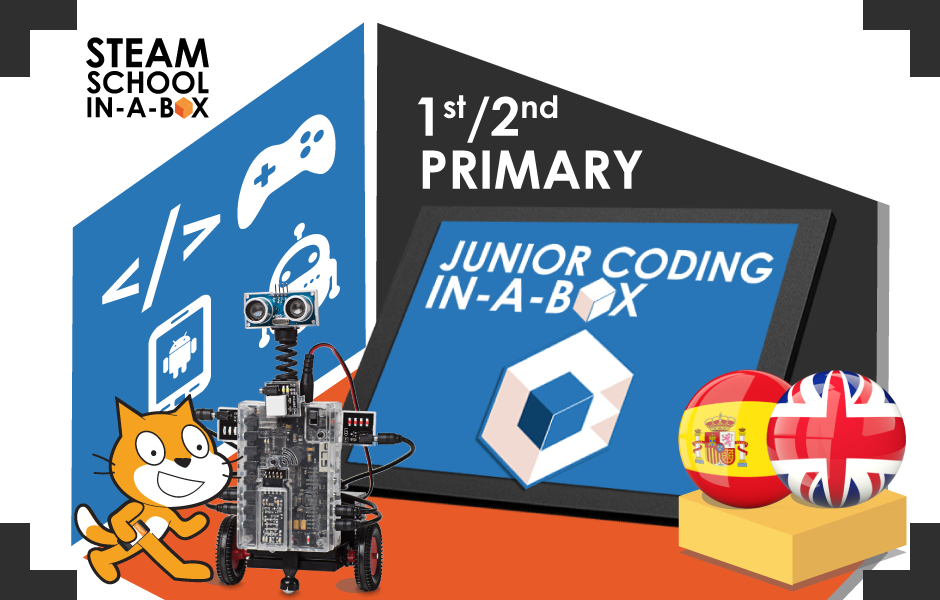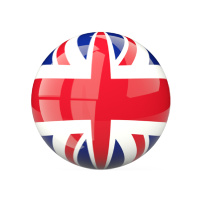### 1ST & 2ND PRIMARY: PROGRAMMING AND ELECTRO-ROBOTICSSCHOOL STAGE: Primary (1st/2nd)

LANGUAGES:ESTIMATED TEACHING HOURS: 58

TECHNOLOGIES: Scratch, Arduino

CURRICULAR AND KEY COMPETENCES:
BOCM: DECRETO 89/2014

COURSE ACCREDITATION:

In the full course they will be getting badges as the reach the objectives of the course.

### DESCRIPTION

Students will work on mathematical concepts of the first cycle of Primary through programming and electronics with Scratch and Arduino.

Through fun projects such as “Zapataraña” or “Digital Clock”, they will learn to use important programming elements such as loops and variables, while working on mathematical skills such as summation logarithm, mental calculation, time measurement or geometry.

In addition, they will expand programming knowledge by starting in electronics with Arduino, making simple real circuits and using components such as breadboards, LEDs and push buttons.

### MATHEMATICAL CURRICULAR COMPETENCES

• Read, write and compare natural numbers up to 99
• It uses and automates standard sum algorithms with and without carried
• Identifies the time in digital clocks and the equivalences between minutes and seconds
• Describes and locates the situation of an object using spatial concepts
• Identifies, classifies and describes rectangular, square, and triangular geometric shapes, using the appropriate geometric vocabulary
• Correctly sorts random and non-random events

### TECHNOLOGICAL AIMS

• PROGRAMMING OF VIDEO GAMES ORIENTED TO THE MATHEMATICS
• Easily use the different basic tools of the programming environment
• Places and moves objects in a given direction
• Starts and stops running a program
• Handles with ease the main groups of blocks in the environment
• It modifies, through editing, the appearance of objects. Create new objects: actors, backgrounds and sounds
• Properly uses messages, variables and lists
• Use costumes
• Working with conditionals
• Use comparative and mathematical operators correctly
• Use mathematical operations
• Understand how loops work
• Draw with the pencil tool
• Use keyboard events
• ROBOTICS
• Understand how block programming works with Ardublock
• Differentiate electronic devices: actuators, sensors and others
• Learn the concept of variable
• Working with conditionals
• Use different types of operators
• Understanding and controlling servos